# 卷积与转置卷积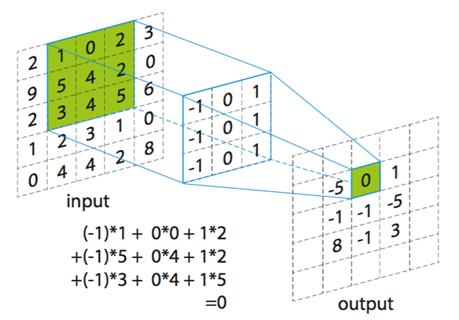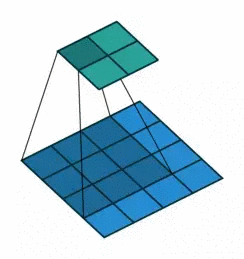• 输入矩阵可以展开成维数为 [16, 1] 的矩阵，记作 $x$
• 输出矩阵可以展开成维数为 [4, 1] 的矩阵，记作 $y$
• 卷积核可以表示为 [4, 16] 的矩阵，记作 C，其中非 0 的值表示卷积对应的第 i 行 j 列的权重。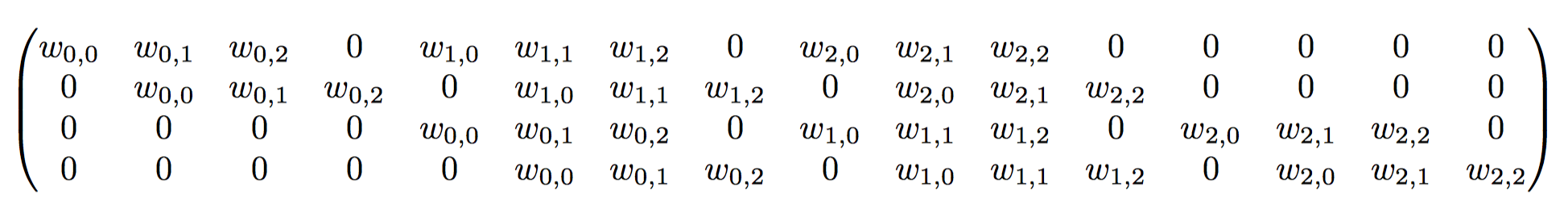• 所以卷积可以用 $y = C * x = [4, 16] * [16, 1]=[4, 1]$来表示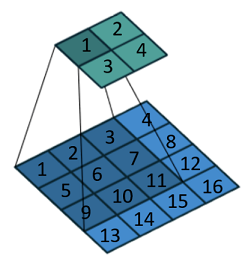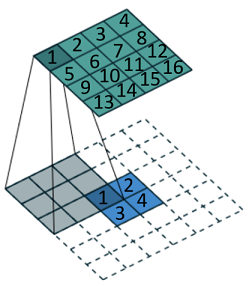# coding:utf-8
from PIL import Image
import numpy as np
import torchvision.transforms as transforms
import torch
from torch import nn

x=Image.open("demo.jpg")
x.show()
x=transforms.ToTensor()(x)
x=torch.unsqueeze(x,0)#添加一个维度
conv_trans = nn.ConvTranspose2d(3, 3,(12,12), 2)# 定义转置卷积
y=conv_trans(x)#转置卷积计算
y=torch.squeeze(y)#扔掉一个维度
#结果显示
imgout=transforms.ToPILImage()(y)
print(x.shape)
print(y.shape)
imgout.show()https://www.jianshu.com/p/09ea4df7a788?utm_source=oschina-app

https://cloud.tencent.com/developer/article/1363619

### 手机扫码访问

•